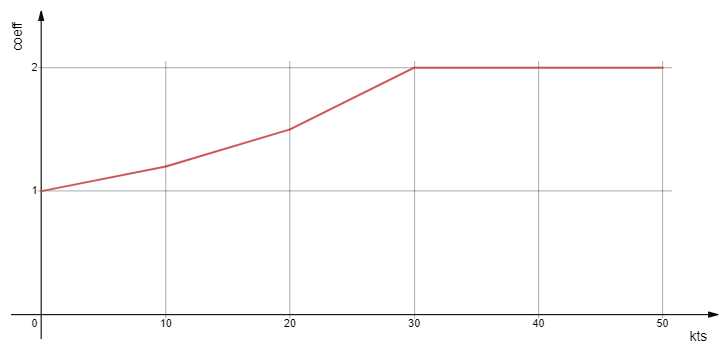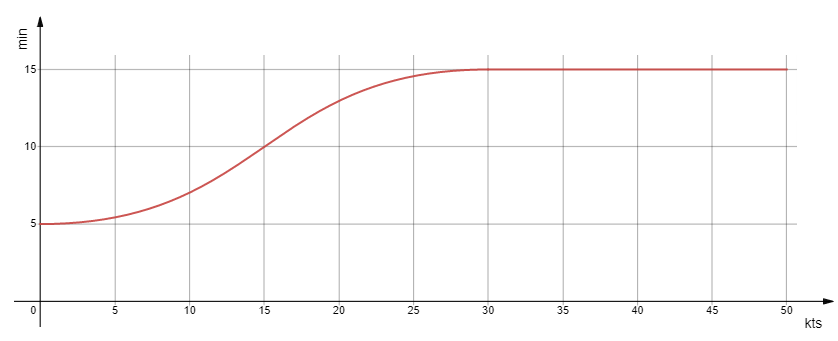### Calculation of energy losses and recovery times

As in reality, the maneuvers and changes of sail are more or less tiring in Virtual Regatta, depending on the strength of the wind and the boat used..

On this page, you can find different information relating to this functionality, in particular concerning the consumption of energy or stamina for the different types of maneuvers.

ManeuverLoss rate
Tack10%
Gybe10%
Sail change20%

Moreover, the influence of the force of the wind is given to us by the following curve :In general, the loss of energy $P_l$ is equal to :

• at the loss rate of the basic maneuver $P_{e_{Base}}$if the wind speed (TWS) is equal to 0 knts,
• to the result of the following calculation : $$P_l(tws) ={ P_{l_{Base}} \times f_l(tws) \times C_{boat}}$$ Avec :
• $P_{l_{Base}}$ : Maneuver Default Loss Rate
• $f_l(tws)$ : Normalized contribution from 1 to 2 of the reference curve of energy losses
• $C_{boat}$ : Boat multiplier from 1 to 2

The influence of the wind on the loss of energy or stamina is given to us by the following curve:This curve can be broken down into 4 sections.

• From 0 to 10 knts $$f_l(tws) = {0.02 \times tws + 1}$$
• From 10 to 20 knts $$f_l(tws) = {0.03 \times tws + 0.9}$$
• From 20 to 30 knts $$f_l(tws) = {0.05 \times tws + 0.5}$$
• Superior to 30 knts $$f_l(tws) = {2}$$

As on a real boat, the type of boat affects the time required to perform a maneuver.

To date, Virtual Regatta has not officially communicated the coefficient for each boat.

Nevertheless, thanks to the effort of the community, we know that there are 4 different coefficients :

$\times 1$ , $\times 1,2$ , $\times 1,5$ , $\times 2$

After testing, the table below seems to achieve consensus but cannot be guaranteed.

BoatCoefficient
Figaro 31
Class 401
Imoca1,2
Mini 6.501
Ultim (solo)1,5
Ultim (crew)1,5
Ocean 501
Caravelle2
Super Maxi 1001,5
Offshore Racer1

As in reality, the calmer the sailing conditions, the faster your skipper will recover his energy.

On this page, you can find different information relating to this functionality, in particular relating to the recovery of energy or stamina.

The influence of the force of the wind is given to us by the following curve :In general, the time to recover a 1% of energy $T_r$ is equal to :

• $T_r{min}$ = 5 min per point if the wind speed (TWS) is equal to 10 knots,
• $T_r{max}$ = 15 min if the wind speed (TWS) is greater than 30 knots,
• to the result of the following calculation : $$T_r(tws) ={ { { (T_r{max} - T_r{min}) \times f_r(tws)}\over 100}}$$ With :
• $T_r(tws)$ : Recovery time
• $T_r{min}$ : Minimum recovery time
• $T_r{max}$ : Maximum recovery time
• $f_r(tws)$ : Normalized contribution from 0 to 100% of the reference curve

The influence of the wind on the recovery of energy or stamina is given to us by the following curve:It can be seen that this curve has the same appearance as the curve used as the basis for calculating the penalties.

Nevertheless, the curve has undergone a deformation as well as a slippage of the reference point on the abscissa.

ITYC therefore uses the penalty reference curve by applying these transformations .